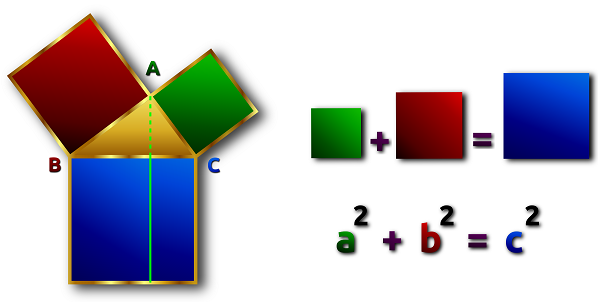# Definition of Pythagoras' TheoremPythagoras' Theorem states that in a right angled triangle, the square of the hypotenuse (the long side) is equal to the sum of the squares of the other two sides.

It can be written as the formula

$a^2 + b^2 = c^2$

This theorem is attributed to Pythagoras, a Greek mathematician and philosopher who founded a cult that studied mathematics and music. This cult had a number of interesting rules, such as never eating beans and never touching a white rooster. However, some Babylonian tablets over a thousand years before Pythagoras' time suggest that the ancient Babylonians had some knowledge of this theorem.

### Description

The aim of this dictionary is to provide definitions to common mathematical terms. Students learn a new math skill every week at school, sometimes just before they start a new skill, if they want to look at what a specific term means, this is where this dictionary will become handy and a go-to guide for a student.

### Audience

Year 1 to Year 12 students

### Learning Objectives

Learn common math terms starting with letter P

Author: Subject Coach
You must be logged in as Student to ask a Question.